## One-sided Limits

For some functions, it is appropriate to look at their behavior from one side only. If x approaches c from the right only, you writeor if x approaches c from the left only, you writeIt follows, then, thatif and only ifExample 1: EvaluateBecause x is approaching 0 from the right, it is always positive;is getting closer and closer to zero, so. Although substituting 0 for x would yield the same answer, the next example illustrates why this technique is not always appropriate.

Example 2: Evaluate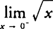.

Because x is approaching 0 from the left, it is always negative, and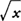does not exist. In this situation,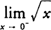DNE. Also, note thatDNE because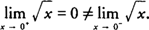.

Example 3: Evaluate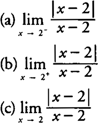a. As x approaches 2 from the left, x − 2 is negative, and | x − 2|=− ( x − 2); hence,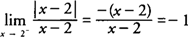b. As x approaches 2 from the right, x − 2 is positive, and | x − 2|= x − 2; hence;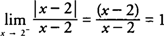c. Because